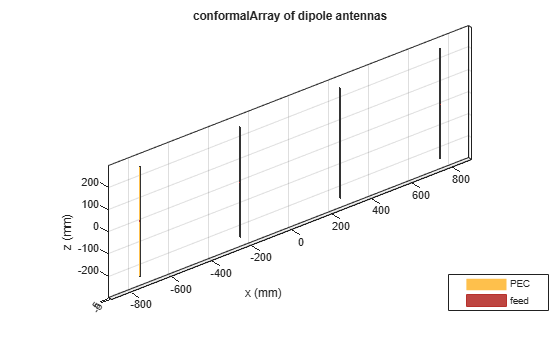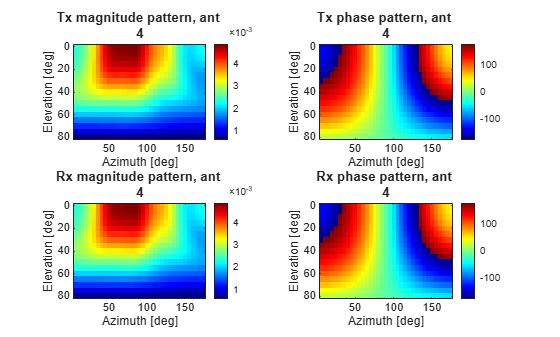Documentation

## Comparison of Antenna Array Transmit and Receive Manifold

This example calculates and compares the transmit and receive manifolds for a basic half-wavelength dipole antenna array. The array manifold is a fundamental property of antenna arrays, both in transmit and receive configurations. The transmit and receive manifolds are theoretically the same due to the reciprocity theorem. This example validates this equality thus providing an important verification of the calculations performed by the Antenna Toolbox™.

### Analysis Setup

Define the variables necessary for calculating the transmit and receive manifolds of an antenna. The frequency for the analysis is 300 MHz which results in a free-space wavelength of approximately 1. Define the sweep angles for azimuth and elevation. These variables will be used to calculate the positions in the far-field of the antenna at which the electric and magnetic fields are computed.

```fc = 3e8; lambda = physconst('lightspeed')/fc; R = 100*lambda; M = 4; ZL = []; phi = 5:5:175; psi = 0:5:80; nphi = length(phi); npsi = length(psi); np = nphi*npsi; PHI = kron(ones(1,npsi),pi/180*phi); PSI = kron(pi/180*psi,ones(1,nphi));```

### Create Cartesian Co-ordinates for Observation and Unit Vectors

The azimuth and elevation angle variables are used to calculate the points on a sphere with radius of $100\lambda$ and the unit vectors.

```antennapos = [[-(M-1)/2:(M-1)/2]*lambda/2;zeros(2,M)]'; x = R*cos(PSI).*cos(PHI); y = R*cos(PSI).*sin(PHI); z = R*sin(PSI); Points = [x;y;z]; h = [-sin(PHI);cos(PHI);zeros(size(PHI))]; v = [-cos(PHI).*sin(PSI);-sin(PHI).*sin(PSI);cos(PSI)]; u = [cos(PHI).*cos(PSI);sin(PHI).*cos(PSI);sin(PSI)];```

### Create Antenna Array

Create a half-wavelength dipole antenna, positioned vertically along the z-axis. Use this element to build a conformal array of dipoles arranged in a linear configuration with half-wavelength spacing between elements.

```d = dipole('Length',lambda/2,'Width',lambda/200); elem = [d,d,d,d]; dA = conformalArray('Element',elem,'ElementPosition',antennapos); figure; show(dA)```### Compute Array Transmit Manifold

Use EHfields to calculate the electric and magnetic field vectors in the transmit mode for the array. The field calculation at 300 MHz is done at the observation points specified on the far-field sphere and considering the vertical polarization contribution explicitly since the dipole orientation is vertical. The analysis is conducted such that the element under excitation has a $50\Omega$ load in series with the voltage source, and all other elements, which are not under excitation, do not have any internal impedance.

```nomLoad = lumpedElement('Impedance',ZL); actLoad = lumpedElement('Impedance',50); for i=[1,1:M] % setup loads for m=1:M dA.Element(m).Load = nomLoad; end dA.Element(i).Load = actLoad; % setup active element ampTaper = zeros(1,M); ampTaper(i) = 1; dA.AmplitudeTaper = ampTaper; [E,H] = EHfields(dA,fc,Points); Etx(i,:) = sum(E.*v); end```

### Compute Array Receive Manifold

To calculate the receive manifold, consider a plane wave incident upon the array with the same electric field polarization but opposite in direction to the transmit mode. Use the planeWaveExcitation object and the feedCurrent function to compute the current passing through the feeds in response to the impinging plane wave.

```nomLoad = lumpedElement('Impedance',ZL); for m=1:M dA.Element(m).Load = nomLoad; end for n = 1:np dirVec = -u(:,n); polVec = v(:,n); p = planeWaveExcitation('Element',dA,'Direction',dirVec,'Polarization',polVec); Erx(:,n) = feedCurrent(p,fc).'; end```

### Compute Normalized Error Between Manifolds

Calculate the normalized error between the transmit and receive manifolds for each element in the array. Reshape the error to plot as a function of the azimuth and elevation angles respectively

```for i=1:M a(i) = Erx(i,:)/Etx(i,:); err(i,:) = abs(Erx(i,:)-a(i)*Etx(i,:)); mse = sqrt(mean(abs(a(i)*Etx(i,:)).*abs(Erx(i,:)))); err(i,:) = err(i,:)/mse; Etx(i,:) = a(i)*Etx(i,:); end ETX = reshape(Etx,M,nphi,npsi); ERX = reshape(Erx,M,nphi,npsi);```
```for i = 1:M ETXmag(i,:,:) = abs(squeeze(ETX(i,:,:)))'; ETXphase(i,:,:) = 180/pi*angle(squeeze(ETX(i,:,:)))'; ERXmag(i,:,:) = abs(squeeze(ERX(i,:,:)))'; ERXphase(i,:,:) = 180/pi*angle(squeeze(ERX(i,:,:)))'; end ERR = 20*log10(reshape(mean(err),nphi,npsi));```

### Plot the Transmit, Receive Manifold and the Error Pattern

The transmit and receive manifolds show a great degree of similarity in the magnitude and phase plots. This is confirmed by the error plot with a maximum error of approximately -40 dB over the span of azimuth and elevation angles.

```for i=1:M figure; subplot(221) imagesc(phi,psi,squeeze(ETXmag(i,:,:))); colorbar colormap('jet') xlabel('Azimuth [deg]','LineWidth',6); ylabel('Elevation [deg]','LineWidth',6); title(['Tx magnitude pattern, ant ',num2str(i)],'FontSize',10) subplot(222) imagesc(phi,psi,squeeze(ETXphase(i,:,:))); colorbar colormap('jet') xlabel('Azimuth [deg]','LineWidth',6); ylabel('Elevation [deg]','LineWidth',6); title(['Tx phase pattern, ant ',num2str(i)],'FontSize',10) subplot(223) imagesc(phi,psi,squeeze(ERXmag(i,:,:))); colorbar colormap('jet') xlabel('Azimuth [deg]','LineWidth',6); ylabel('Elevation [deg]','LineWidth',6); title(['Rx magnitude pattern, ant ',num2str(i)],'FontSize',10); subplot(224) imagesc(phi,psi,squeeze(ERXphase(i,:,:))); colorbar colormap('jet') xlabel('Azimuth [deg]','LineWidth',6); ylabel('Elevation [deg]','LineWidth',6); title(['Rx phase pattern, ant ',num2str(i)],'FontSize',10); end``````figure; imagesc(phi,psi,ERR'); colorbar colormap('jet') xlabel('Azimuth [deg]','LineWidth',6); ylabel('Elevation [deg]','LineWidth',6); title('Normalized error','FontSize',10)```### Acknowledgement

This example was developed in collaboration with Prof. Benjamin Friedlander at the University of California, Santa Cruz.